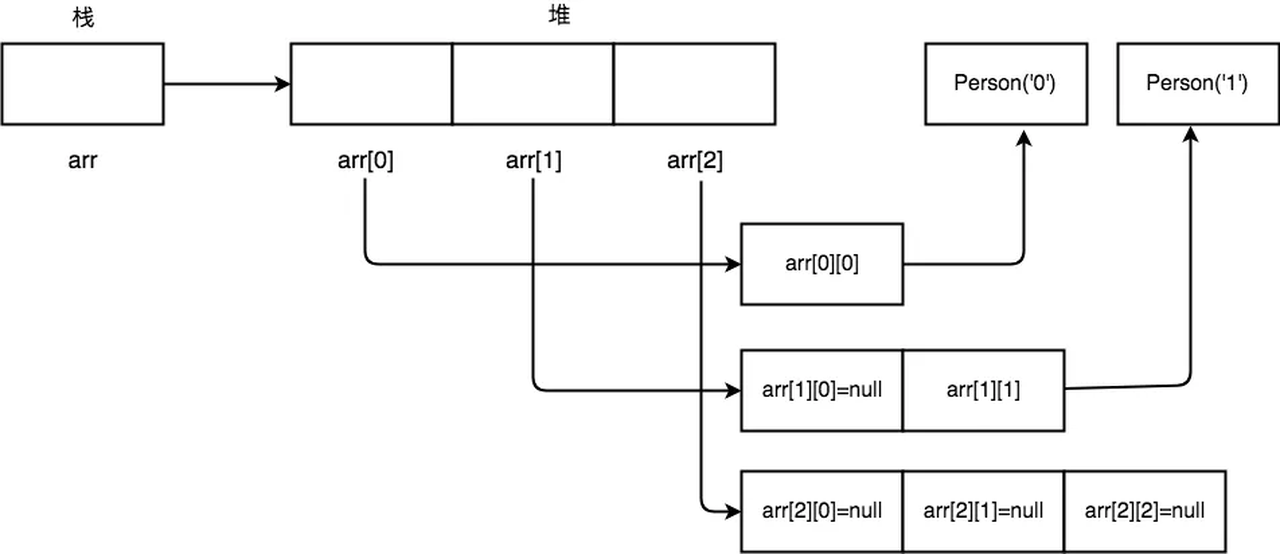# 详解数据结构中的“数组”与编程语言中的“数组”的区别和联系

``````var arr = new Array(4,'hello', new Date());

``````int arr[][] = new int[];
arr = new int;
arr = new int;
arr = new int;

### 1. C/C++中数组的实现方式

C/C++中的数组，是非常标准的数据结构中的数组，也就是连续存储相同类型的数据的一块内存空间。在C/C++中，不管是基本类型数据，比如int、long、char，还是结构体、对象，在数组中都是连续存储的。我举了一下例子，你可以看下。

``````int arr;
arr = 0;
arr = 1;
arr = 2;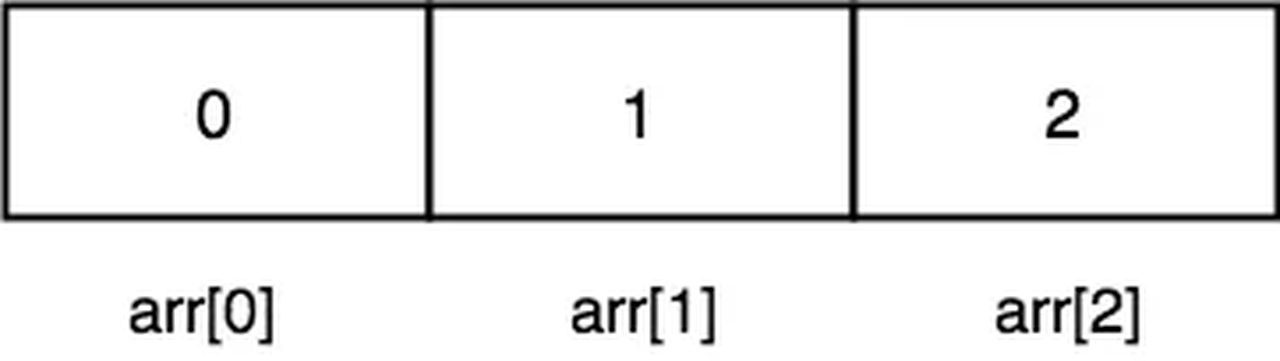``````struct Dog {
char a;
char b;
};
struct Dog arr;
// 为了节省页面，放到了一行里了
arr.a = '0'; arr.b = '1';
arr.a = '2'; arr.b = '3';
arr.a = '4'; arr.b = '5';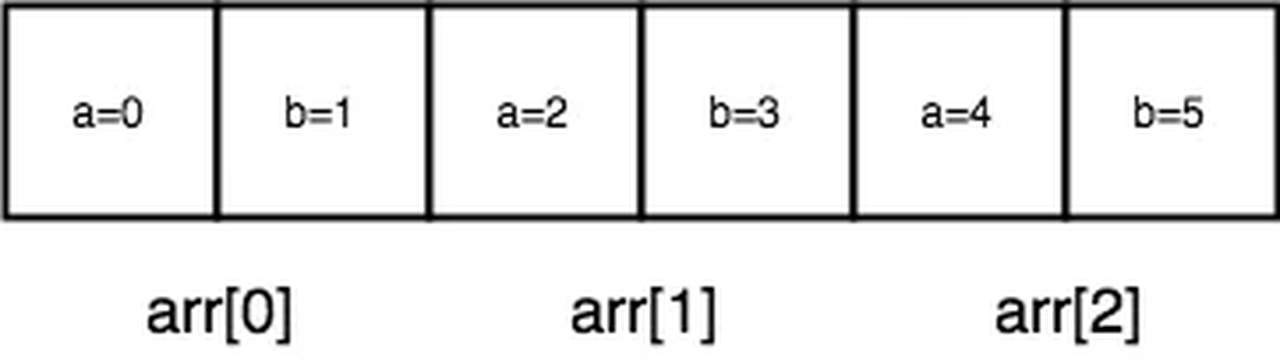``````struct Dog {
char a;
char b;
};
struct Dog arr;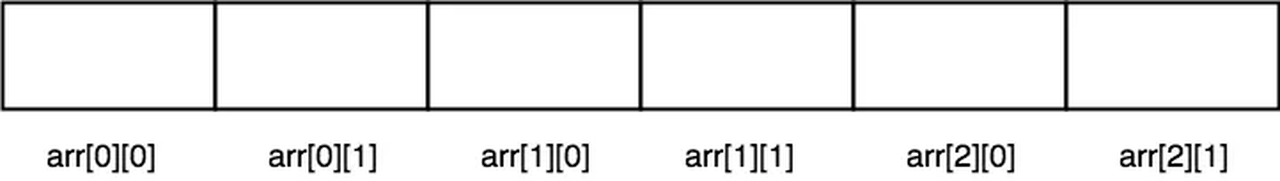### 2. Java中数组的实现方式

``````int arr[] = new int;
arr = 1;
arr = 2;
arr = 3;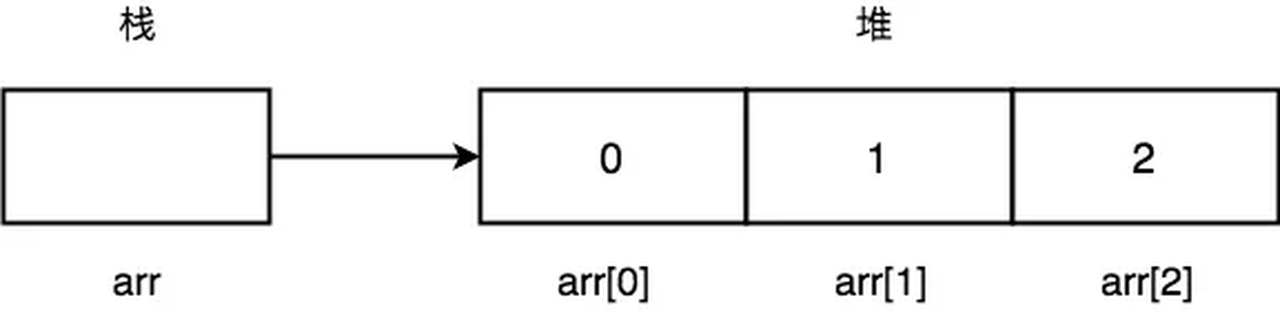``````class Person {
private String name;
public Person(String name) {
this.name = name;
}
}

Person arr[] = new Person;
arr = new Person("0");
arr = new Person("1");
arr = new Person("2");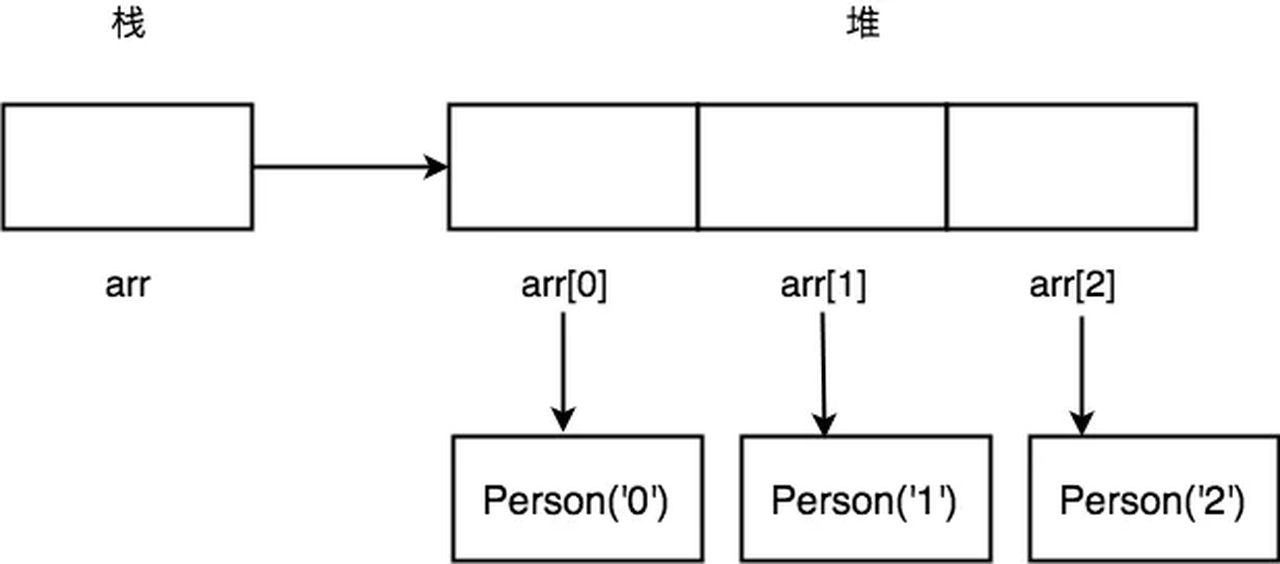Java中的二维数组，跟数据结构中二维数组，有很大区别。在Java中，二维数组中的第二维，可以是不同长度的。这句话有点不好理解。我举个例子说明一下。

``````int arr[][] = new int[];
arr = new int;
arr = new int;
arr = new int;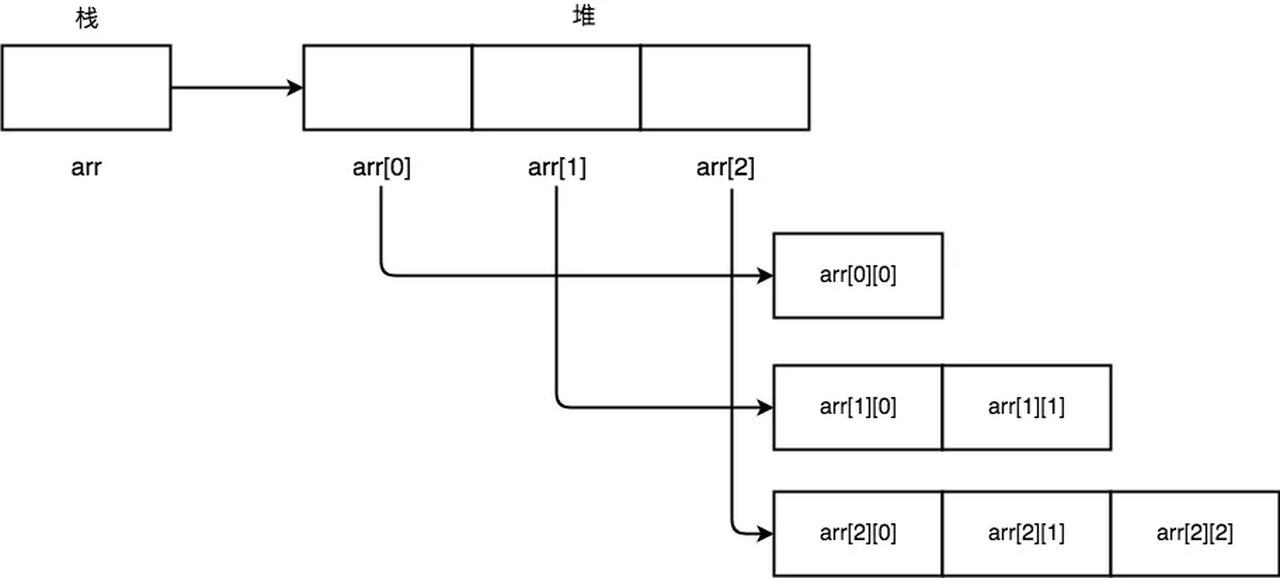``````Person arr[][] = new Person[];
arr = new Person;
arr = new Person;
arr = new Person;

arr = new Person("0");
arr = new Person("1");